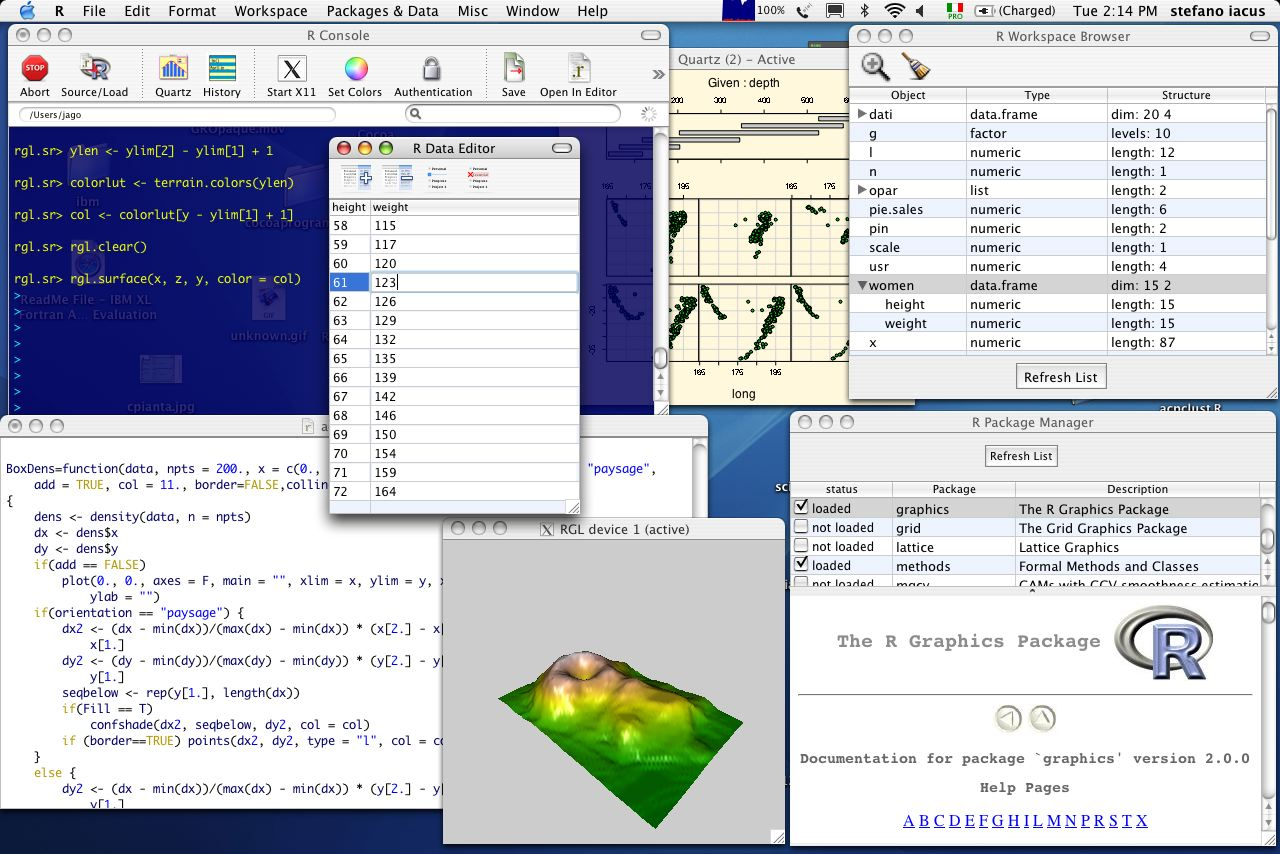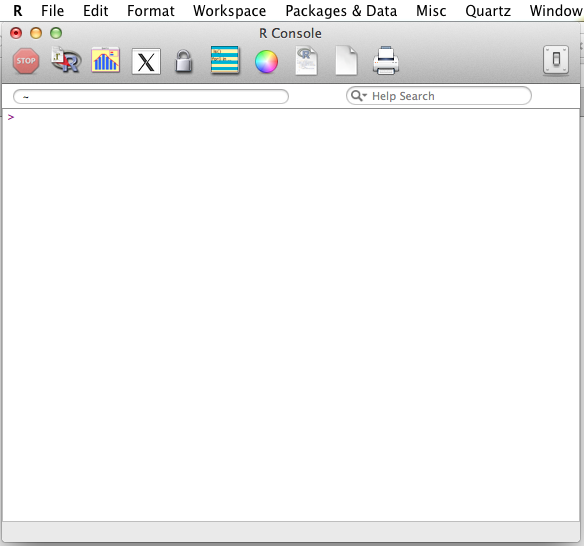# r Programming Language for Statistical Analysis

It is becoming important for today’s finance professionals to have the basic programming skills as more and more of their work involves building statistical and financial models. One such programming language is r which is fast becoming a programming language of choice for statistical analysis.

What is r?

R is a free software environment for statistical computing and graphics.

It is an environment within which statistical techniques are implemented.

What can r be used for?

R can be used for a variety of data analysis problems. R provides a wide variety of statistical (linear and nonlinear modelling, classical statistical tests, time-series analysis, classification, clustering, ...) and graphical techniques, and is highly extensible.

Broadly, it can be used for purposes such as:

• Data Manipulation

• Connecting to data sources

• Slicing and dicing data

• Modeling and Computation

• Statistical modeling

• Numerical simulation

• Data Visualization

• Visualizing fit for models

• Composing statistical graphics

One of R's strengths is the ease with which well-designed publication-quality plots can be produced, including mathematical symbols and formulae where needed. Great care has been taken over the defaults for the minor design choices in graphics, but the user retains full control.What platforms can it run on?

It compiles and runs on a wide variety of UNIX platforms, Windows and MacOS.

The R Application

Let’s launch the R application and take a look at its GUI. The GUI for R is essentially a command prompt with a few menu bars. It looks slightly different on different operating systems. On Mac OS it looks as shown below:At the command prompt, you can type your command to execute it.

Let’s take a look at a few examples to get an idea of what we can do. In the future articles we will go in depth into the programming.

Example 1:

This is, perhaps an extremely simple example, where we show how you can use it as a calculator.

Suppose you want to find out the result of the following:

\= 500 * (230 – 145)

You can simply write this at the r command prompt and press enter to get the result. The result will be shown on the next line. > 500*(230-145)  42500

Example 2: